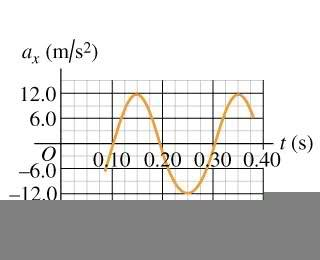# Simple Harmonic Motion of a bolt of mass

• QuarkCharmer

## Homework Statement

A bolt of mass 2.10×10^-2 kg moves with SHM that has an amplitude of 0.245 m and a period of 1.495 s. The displacement of the bolt is + 0.245 m when t=0.

A.) Find the displacement of the bolt at time t=0.500s

## The Attempt at a Solution

Part A.)
$$ω= \frac{2π}{1.495}$$
$$ω= 4.20$$

Since the function starts at +0.245 I modeled the motion by this:
$$x(t) = Acos(ωt + \phi)$$
$$x(t) = 0.245cos(4.20t)$$

I then evaluated x(t) at t = .5 and I got that:
$$x(.5) = -.123$$

Make sense?

(There are more parts to this problem, but I wanted to make sure I remember graphing of trig functions correctly.)

Make sense?

Perfectly!Perfectly!Great! (Also, Happy Halloween ILS)

Now I am stuck with this one:

On a frictionless, horizontal air track, a glider oscillates at the end of an ideal spring of force constant 4.00 N/cm. The graph in the figure shows the acceleration of the glider as a function of time.

k = 400 N/m

I found the mass of the glider to be 0.405 kg.Find the maximum displacement of the glider from the equilibrium point.
I don't understand how this is possible, considering it's a graph of acceleration vs. time. I lose information in the form of the constant of integration? Can you point me in the right direction on this part?

Alas, no Halloween where I live! :grumpy:

How did you find the mass of the glider?
Anyway, with it you can use F=kx=ma to find the amplitude.

Alternatively, SHM has x=A cos wt (starting from some irrelevant time in this case).
Take the derivative twice and you get the equation for acceleration a.
You should be able to use the information in the graph to figure out A.

Alas, no Halloween where I live! :grumpy:

How did you find the mass of the glider?
Anyway, with it you can use F=kx=ma to find the amplitude.

Alternatively, SHM has x=A cos wt (starting from some irrelevant time in this case).
Take the derivative twice and you get the equation for acceleration a.
You should be able to use the information in the graph to figure out A.

I can read the amplitude (of acceleration) from the graph, and integrate the function into:
$$\upsilon(t)=\frac{1.2}{\pi}cos(10\pi t) + C$$
But I can't manage to get an initial condition (or whatever you call it in this case) where I can solve for the constant and continue to integrate.

Edit: Or can I say that since velocity is zero when acceleration is at it's minimum, (and acceleration hits a min at t=.25), then v(.25) = 0, and thus C = 0?

I'll see what I can put together with F=ma.

$$F = -kx = ma$$
$$-kx = ma$$
$$-(400)x = (0.405)(12)$$
$$x_{amplitude} = 0.01215 m$$

$$0.01215 m \frac{100cm}{1m} = 1.215 cm$$
1.22 cm is the Amplitude of displacement vs time?

If that is correct, then:
"Find the maximum force the spring exerts on the glider. "
Would simply be:
$$F = ma$$
$$F = (0.405)(12)$$ (assuming force is max when accel is max)
$$F = 4.86 N$$

That just seems like a low number?

Last edited:
But it's correct!

Thank you!

Edit: Oh and I found the glider mass by reading the period off the acceleration/time graph and then applying:
$$T = 2 \pi \sqrt{\frac{m}{k}}$$

Last edited:
I can read the amplitude (of acceleration) from the graph, and integrate the function into:
$$\upsilon(t)=\frac{1.2}{\pi}cos(10\pi t) + C$$
But I can't manage to get an initial condition (or whatever you call it in this case) where I can solve for the constant and continue to integrate.

Edit: Or can I say that since velocity is zero when acceleration is at it's minimum, (and acceleration hits a min at t=.25), then v(.25) = 0, and thus C = 0?

Yep!But it's correct!

Thank you!

Edit: Oh and I found the glider mass by reading the period off the acceleration/time graph and then applying:
$$T = 2 \pi \sqrt{\frac{m}{k}}$$

Aha!